### Example 64.2 Probit-Normal Model with Binomial Data

For this example, consider the data from Weil (1970), also studied by: Williams (1975); Ochi and Prentice (1984); McCulloch (1994). In this experiment 16 pregnant rats receive a control diet and 16 receive a chemically treated diet, and the litter size for each rat is recorded after 4 and 21 days. The SAS data set follows:

data rats;
input trt \$ m x @@;
if (trt='c') then do;
x1 = 1;
x2 = 0;
end;
else do;
x1 = 0;
x2 = 1;
end;
litter = _n_;
datalines;
c 13 13   c 12 12   c  9  9   c  9  9   c  8  8   c  8  8   c 13 12   c 12 11
c 10  9   c 10  9   c  9  8   c 13 11   c  5  4   c  7  5   c 10  7   c 10  7
t 12 12   t 11 11   t 10 10   t  9  9   t 11 10   t 10  9   t 10  9   t  9  8
t  9  8   t  5  4   t  9  7   t  7  4   t 10  5   t  6  3   t 10  3   t  7  0
;


Here, m represents the size of the litter after 4 days, and x represents the size of the litter after 21 days. Also, indicator variables x1 and x2 are constructed for the two treatment levels.

Following McCulloch (1994), assume a latent survival model of the form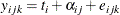where i indexes treatment, j indexes litter, and k indexes newborn rats within a litter. The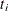represent treatment means, the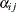represent random litter effects assumed to be iid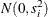, and the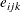represent iid residual errors, all on the latent scale.

Instead of observing the survival times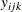, assume that only the binary variable indicating whetherexceeds 0 is observed. If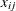denotes the sum of these binary variables for the ith treatment and the jth litter, then the preceding assumptions lead to the following generalized linear mixed model: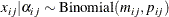where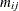is the size of each litter after 4 days and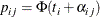The PROC NLMIXED statements to fit this model are as follows:

proc nlmixed data=rats;
parms t1=1 t2=1 s1=.05 s2=1;
eta = x1*t1 + x2*t2 + alpha;
p   = probnorm(eta);
model x ~ binomial(m,p);
random alpha ~ normal(0,x1*s1*s1+x2*s2*s2) subject=litter;
estimate 'gamma2' t2/sqrt(1+s2*s2);
predict p out=p;
run;


As in Example 64.1, the PROC NLMIXED statement invokes the procedure and the PARMS statement defines the parameters. The parameters for this example are the two treatment means, t1 and t2, and the two random-effect standard deviations, s1 and s2.

The indicator variables x1 and x2 are used in the program to assign the proper mean to each observation in the input data set as well as the proper variance to the random effects. Note that programming expressions are permitted inside the distributional specifications, as illustrated by the random-effects variance specified here.

The ESTIMATE statement requests an estimate of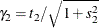, which is a location-scale parameter from Ochi and Prentice (1984).

The PREDICT statement constructs predictions for each observation in the input data set. For this example, predictions of p and approximate standard errors of prediction are output to a SAS data set named P. These predictions are functions of the parameter estimates and the empirical Bayes estimates of the random effects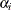.

The output for this model is as follows.

Output 64.2.1: Specifications, Dimensions, and Starting Values

The NLMIXED Procedure

Specifications
Data Set WORK.RATS
Dependent Variable x
Distribution for Dependent Variable Binomial
Random Effects alpha
Distribution for Random Effects Normal
Subject Variable litter
Optimization Technique Dual Quasi-Newton

Dimensions
Observations Used 32
Observations Not Used 0
Total Observations 32
Subjects 32
Max Obs Per Subject 1
Parameters 4

Parameters
t1 t2 s1 s2 NegLogLike
1 1 0.05 1 54.9362323

The Specifications table provides basic information about this nonlinear mixed model (Output 64.2.1). The Dimensions table provides counts of various variables. Note that each observation in the data comprises a separate subject. Using the starting values in the Parameters table, PROC NLMIXED determines that the log-likelihood function can be approximated with sufficient accuracy with a seven-point quadrature rule.

Output 64.2.2: Iteration History for Probit-Normal Model

Iteration History
Iter   Calls NegLogLike Diff MaxGrad Slope
1   2 53.9933934 0.942839 11.03261 -81.9428
2   3 52.875353 1.11804 2.148952 -2.86277
3   5 52.6350386 0.240314 0.329957 -1.05049
4   6 52.6319939 0.003045 0.122926 -0.00672
5   8 52.6313583 0.000636 0.028246 -0.00352
6   11 52.6313174 0.000041 0.013551 -0.00023
7   13 52.6313115 5.839E-6 0.000603 -0.00001
8   15 52.6313115 9.45E-9 0.000022 -1.68E-8

 NOTE: GCONV convergence criterion satisfied.

The Iteration History table indicates successful convergence in 8 iterations (Output 64.2.2).

Output 64.2.3: Fit Statistics for Probit-Normal Model

Fit Statistics
-2 Log Likelihood 105.3
AIC (smaller is better) 113.3
AICC (smaller is better) 114.7
BIC (smaller is better) 119.1

The Fit Statistics table lists useful statistics based on the maximized value of the log likelihood (Output 64.2.3).

Output 64.2.4: Parameter Estimates for Probit-Normal Model

Parameter Estimates
Parameter Estimate Standard Error DF t Value Pr > |t| Alpha Lower Upper Gradient
t1 1.3063 0.1685 31 7.75 <.0001 0.05 0.9626 1.6499 -0.00002
t2 0.9475 0.3055 31 3.10 0.0041 0.05 0.3244 1.5705 9.283E-6
s1 0.2403 0.3015 31 0.80 0.4315 0.05 -0.3746 0.8552 0.000014
s2 1.0292 0.2988 31 3.44 0.0017 0.05 0.4198 1.6385 -3.16E-6

The Parameter Estimates table indicates significance of all the parameters except S1 (Output 64.2.4).

The Additional Estimates table displays results from the ESTIMATE statement (Output 64.2.5). The estimate of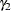equals 0.66, agreeing with that obtained by McCulloch (1994). The standard error 0.22 is computed using the delta method (Billingsley, 1986; Cox, 1998).
Not shown is the P data set, which contains the original 32 observations and predictions of the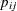.